Courses

# Theory Of Columns - 1

## 10 Questions MCQ Test Mock Test Series for Civil Engineering (CE) GATE 2020 | Theory Of Columns - 1

Description
This mock test of Theory Of Columns - 1 for Civil Engineering (CE) helps you for every Civil Engineering (CE) entrance exam. This contains 10 Multiple Choice Questions for Civil Engineering (CE) Theory Of Columns - 1 (mcq) to study with solutions a complete question bank. The solved questions answers in this Theory Of Columns - 1 quiz give you a good mix of easy questions and tough questions. Civil Engineering (CE) students definitely take this Theory Of Columns - 1 exercise for a better result in the exam. You can find other Theory Of Columns - 1 extra questions, long questions & short questions for Civil Engineering (CE) on EduRev as well by searching above.
QUESTION: 1

### For a circular column having its ends hinged, the slenderness ratio is 160. The l/d ratio of the column is

Solution: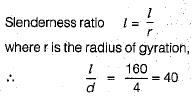QUESTION: 2

### For the case of slender column of length 'l’ and flexural rigidity EI built in at its base and free at the top, the Euler's buckling load is

Solution: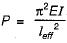For a column fixed at base and free at the top,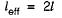∴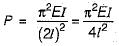QUESTION: 3

### Rankine-GordonTormulafor buckling is valid for

Solution:
QUESTION: 4

The resultant cuts the base of a circular column of diameter ‘d’ with an eccentricity equal to one-fourth of 'd'. The ratio between the maximum compressive stress and the maximum tensile stress is

Solution:

For a solid circular section, the maximum eccentricity for no tension is d/8.
The stress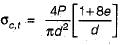for e = d/4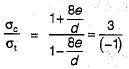The minus sign is due to opposite nature of forces.

QUESTION: 5

A column of length 2.4 m area of cross-section 2,000 mm2 andmoment of inertia of Ixx = 720 x 104 mm4 and Iyy = 80 x 104 mm4 is subjected to buckling load. Both the ends of the column are fixed. What is the slenderness ratio of column?

Solution:

Slenderness ratio of column is given by,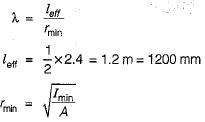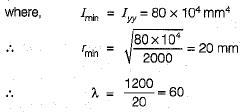QUESTION: 6

A hollow circular column, has D = 100 mm, d = 80 mm. What is its radius of gyration

Solution: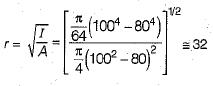QUESTION: 7

If diameter of a long column is reduced by 20%, the percentage reduction in Euler’s buckling load is

Solution:

Euler buckling load P is given by,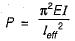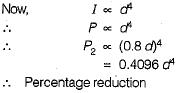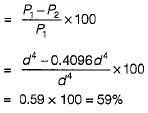QUESTION: 8

A rectangular bar as shown in the figure below carries a tensile load of 1 kN as shown in the figure. Under this load the maximum value of stress over the mean value will increase by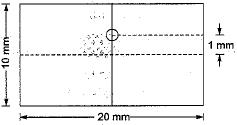Solution:

Mean value of stress,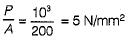Maximum intensity of stress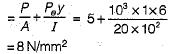∴ Increase in stress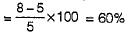QUESTION: 9

A hollow circular column of internal diameter 'd' and external diameter '1.5 d’ is subjected to compressive load. The maximum distance of the point of application of load from the centre for no tension is

Solution: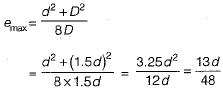QUESTION: 10

Given that
PE = The crippling load given by Euler
PC = The load at failure due to direct compression
PR = The load in accordance with the Rankine's criterion of failure
PR is given by

Solution: